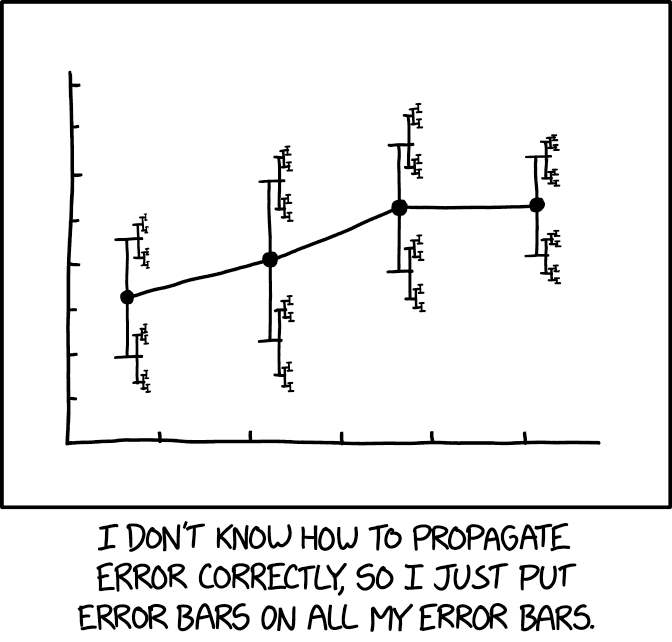## error bars [reposted]

Posted in Books, Kids, pictures, Statistics, University life with tags , , , , , on March 3, 2019 by xi'anA definitely brilliant entry on xkcd that reflects upon the infinite regress of producing error evaluations that are based on estimates. A must for the next class when I introduce error bars and confidence intervals!

## I’m getting the point

Posted in Statistics with tags , , , , , , on February 14, 2019 by xi'anA long-winded X validated discussion on the [textbook] mean-variance conjugate posterior for the Normal model left me [mildly] depressed at the point and use of answering questions on this forum. Especially as it came at the same time as a catastrophic outcome for my mathematical statistics exam.  Possibly an incentive to quit X validated as one quits smoking, although this is not the first attempt

## unbiased estimators that do not exist

Posted in Statistics with tags , , , , , , , on January 21, 2019 by xi'anWhen looking at questions on X validated, I came across this seemingly obvious request for an unbiased estimator of P(X=k), when X~B(n,p). Except that X is not observed but only Y~B(s,p) with s<n. Since P(X=k) is a polynomial in p, I was expecting such an unbiased estimator to exist. But it does not, for the reasons that Y only takes s+1 values and that any function of Y, including the MLE of P(X=k), has an expectation involving monomials in p of power s at most. It is actually straightforward to establish properly that the unbiased estimator does not exist. But this remains an interesting additional example of the rarity of the existence of unbiased estimators, to be saved until a future mathematical statistics exam!

## p-value graffiti in the lift [jatp]

Posted in Statistics with tags , , , , , , , , on January 3, 2019 by xi'an

## a glaringly long explanation

Posted in Statistics with tags , , , , , , , , , , on December 19, 2018 by xi'anIt is funny that, when I am teaching the rudiments of Bayesian statistics to my undergraduate students in Paris-Dauphine, including ABC via Rasmus’ socks, specific questions about the book (The Bayesian Choice) start popping up on X validated! Last week was about the proof that ABC is exact when the tolerance is zero. And the summary statistic sufficient.

This week is about conjugate distributions for exponential families (not that there are many others!). Which led me to explain both the validation of the conjugacy and the derivation of the posterior expectation of the mean of the natural sufficient statistic in far more details than in the book itself. Hopefully in a profitable way.